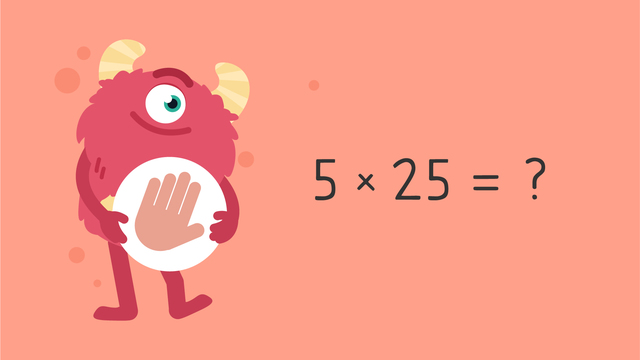# Grams and Kilograms (Word Problems) — Let's Practice!Rating

Ø 5.0 / 1 ratings

The authorsTeam Digital

## Basics on the topicGrams and Kilograms (Word Problems) — Let's Practice!

You can practice grams and kilograms word problems with this video!

### TranscriptGrams and Kilograms (Word Problems) — Let's Practice!

Razzi says get these items ready, because today we're going to practice grams and kilograms - word problems. It's time to begin! Solve this word problem. Josiah has five bouncy balls. The balls weigh twenty-five grams each. What is the total weight of all the balls? Pause the video to work on the problem and press play when you are ready to see the solution! To solve, we need to calculate five multiplied by twenty-five, which is one hundred twenty-five. Add the unit of measurement. Did you also get one hundred twenty-five grams? Let's tackle the next word problem! There is a total of twenty-four kilograms of sand. If all the sand is shared equally into eight bags, how many kilograms of sand are in each bag? Pause the video to work on the problem, and press play when you are ready to see the solution! To solve, we need to calculate twenty-four divided by eight, which is three. Add the unit of measurement. Did you also get three kilograms? Let's tackle the final word problem! A pineapple weighs two hundred fifty grams, and a mango weighs one hundred seventy-seven grams. What is the total weight of the fruits? Pause the video to work on the problem, and press play when you are ready to see the solution! To solve, we need to calculate two hundred fifty plus one hundred-seventy seven, which is four hundred twenty-seven. Add the unit of measurement. Did you also get four hundred twenty-seven grams? Razzi had so much fun practicing with you today! See you next time!